# Rotor Resistance Control of Induction Motor

In 1891 at the Frankfurt exhibition Nikola Tesla presented a crude type of poly phase induction motor. Induction motors are widely used. Nearly 80% of the world’s AC motors are poly phase induction motors. So speed control of this motor is an important agenda. The speed control of poly phase induction motor can be done through the different methods. Here we will discuss about the speed control of wound rotor induction motor using variation of rotor resistance.
The conventional method for variation of rotor resistance was done by inserting a variable rheostat in series with the 3 phase winding of motor. This method of speed control faced problems and this was not economically viable. The variation of rotor resistance can be done by using power electronics devices and this is more efficient method. Here we will show how this can be achieved.

Basic Concept
The torque equation for poly phase induction motor is,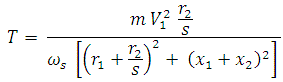Under normal working condition r2/s≫ r1, x1 and therefore the equation becomes,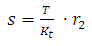This equation shows that for a constant torque, the slip is directly proportional to the rotor resistance. If the resistance in the rotor circuit is increased, the slip is increased and the speed of the rotor is decreased. This method is applicable only to wound rotor induction motor.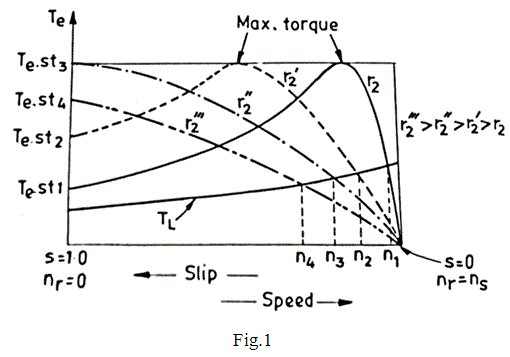From the figure 1 it is clear how does the speed vary with the change of rotor resistance.
At the normal operating condition the slip increases with increasing torque hence they are obeying a linear characteristics. For a fixed load curve, the speed is downward from n1 to n4. From this figure we can also obtain the maximum torque at the starting of the resistance r2”. So, this method has an advantage of achieving maximum torque at the starting period.

Characteristics
But the maximum torque is independent of the rotor resistance as per the equation,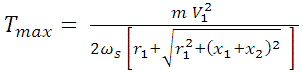This type of speed control is used when the intermittent speed control is required.
It has some drawbacks

1. The rheostat which is used to vary the resistance per phase causes unbalancing in rotor.
2. The resistances generate huge losses and generate heat in the system.
3. In case of a large machine the size of the rheostat will be large and in such case it is not easily portable.
4. It requires more maintenance, hence the cost associated with it is more.
5. This method can not be used for industrial automation purpose since we have to change manually the value of the resistance.

The above mentioned problems can easily be eliminated by using Pulse Width Modulation (PWM), or Pulse Duration Modulation (PDM) with the bridge rectifier and a switching transistor.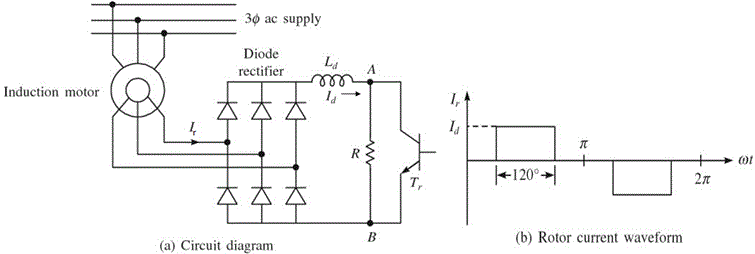Rotor resistance can also be varied steplessly using the circuit shown in figure (a). The AC output voltage of the rotor is rectified by diode bridge rectifier and fed to parallel combination of fixed resistor R and a semiconductor switching transistor Tr. Effective value of resistance across terminal A and B i.e., RAB is varied by varying duty cycle of the transistor Tr, which in turn varies the resistance of the rotor circuit. Inductance is added to the circuit to reduces the ripple and discontinuity in the dc link current Id. Rotor current waveform is shown in the fig.(b) when the ripple is neglected. Thus the rms value of the current will be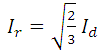Resistance between A and B terminal will be zero when the transistor is on and it will be R when it is off. Therefore average value of resistance between the terminals is given by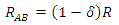Where, δ is the duty ratio of the transistor.
Electrical power consumed by the RAB resistor per phase is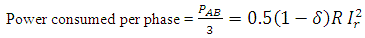From this equation we can suggest that rotor circuit resistance per phase is increased by 0.5(1-δ)R. Thus total circuit resistance can be evaluated.
Therefore the total rotor resistance is varied from Rr to 0.5(1-δ)R as δ is varied from 1 to 0.

### Advantages of Speed Control of Induction Motor Using Static Devices

1. Smooth variations of rotor resistance.
2. Simplicity of operation using close loop control.
3. Quick response of system.
4. Rotor resistance unbalanced can be eliminated using power electronics devices.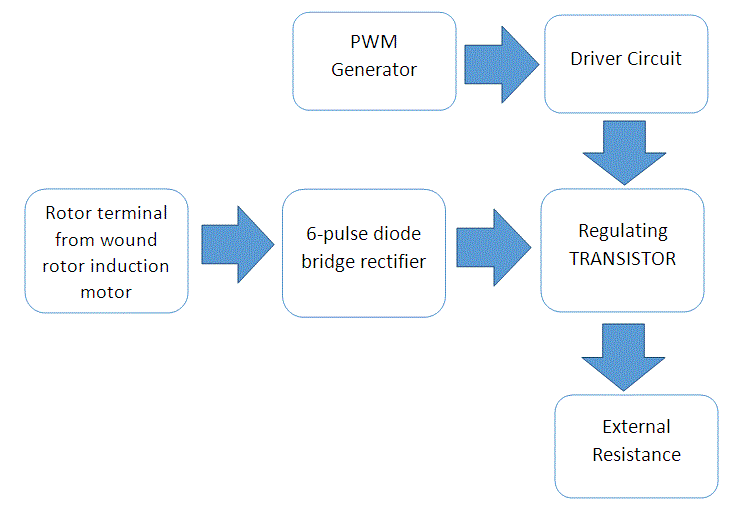Conclusion
Although the method of speed controlling of induction motor using this technology is efficient, but as we are using resistance to control the speed of the motor here also resistive losses occurs, which causes unnecessary heating effect on the motor and also reduces the efficiency in some extent. That is why it cannot be operated for continuous operation, it is used in the intermittent application such as overhead cranes, load fluctuations etc.

Want To Learn Faster? 🎓
Get electrical articles delivered to your inbox every week.
No credit card required—it’s 100% free.